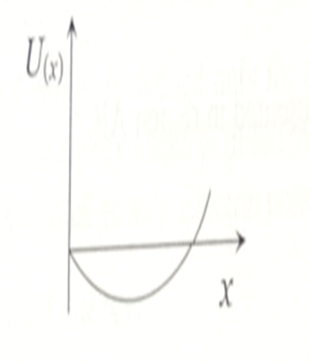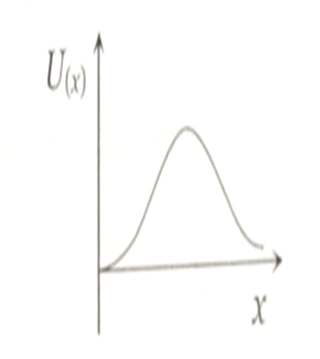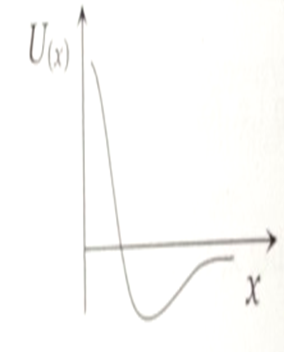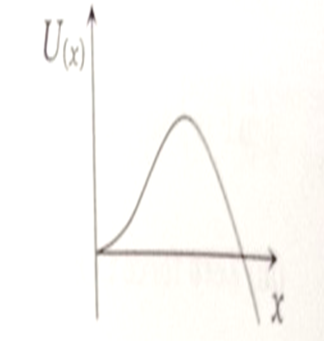NEET Physics Mathematical Tools Questions Solved

A particle which is constrained to move along the x-axis, is subjected to a force in the same direction which varies with the distance x of the particle from the origin as . Here k and a are positive constants. For $x\ge 0$, the functional form of the potential energy ${U}_{\left(x\right)}$ of the particle is

[This question is only for Dropper and XII batch]

1.2.3.4.Concept Videos :-

#16 | Calculus: Differential Equation
#19 | Numericals

Concept Questions :-

Integration
Explanation is a part of a Paid Course. To view Explanation Please buy the course.

Difficulty Level: